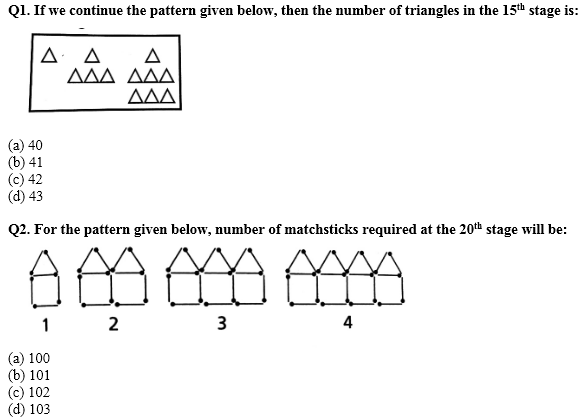Dear Students!!! There is most general as well as a scoring section in all the competitive entrance examinations in the teaching field i.e "Mathematics".Because in this section only one thing is work i.e your accuracy and that could be nourished with the daily practice. So, for this, we are providing you the daily quiz for all teaching exams i.e CTET Exam 2019, DSSSB ,KVS,STET Exam.

Preparing For CTET 2019? Enroll Now For CTET Prime :
Use This Code TEACH5Q3. Fiftieth term of the pattern ABCDABCDABCD ……. is :

(a) A
(b) B
(c) C
(d) D

Q4. If 11 × 11 = 121
111 × 111 = 12231
1111 × 1111 = 1234321
Then 111111 × 111111 will be

(a) 123454321
(b) 12345654321
(c) 132546321
(d) 111 × 111× 111

Q5. Study the pattern below :
7 × 9 = 63
7 × 99 = 693
7 × 999 = 6993
7 × 9,999 = 69993
Continuing this pattern, the product of 7 × 99999 will be :

(a) 6993
(b) 69993
(c) 699993
(d) 6999993

Q6. Study the pattern given below :
9 × 11 = 99
9 × 11 = 1089
999 × 11 = 10989
9999 × 11 = 109989
Continuing the above pattern, the product of 9999999 × 11, without calculating will be :

(a) 109899
(b) 1099899
(c) 10999989
(d) 109999989

Q7. Look at the pattern given below :
(8 – 1) ÷ 7 = 1
(86 – 2) ÷ 7 = 12
(864 – 3) ÷ 7 = 123
(8642 – 4) ÷ 7 = 1234
Continuing this pattern, (86420 – 5) ÷ 7 will be :

(a) 12346
(b) 12364
(c) 12345
(d) 12435Maths Questions for All Teaching Exam :17th April 2019 (Solutions)